# Curvature of a connection

This curvature is also sometimes known as the Riemann curvature tensor. However, the latter term is usually reserved for situations where we have a linear connection, in particular, the Riemann curvature tensor arising from the Levi-Civita connection for a Riemannian or pseudo-Riemannian manifold

## Definition

### Given data

• A connected differential manifold$M$
• A vector bundle$E$ over$M$
• A connection$\nabla$ for$E$

### Definition part

The curvature of$\nabla$ is defined as the map: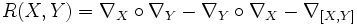$R(X,Y) = \nabla_X \circ \nabla_Y - \nabla_Y \circ \nabla_X - \nabla_{[X,Y]}$

where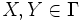$X, Y \in \Gamma$

Note that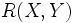$R(X,Y)$ itself outputs a linear map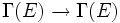$\Gamma(E) \to \Gamma(E)$. We can thus write this as:$R(X,Y)Z = \nabla_X (\nabla_Y Z) - \nabla_Y (\nabla_X Z) - \nabla_{[X,Y]}Z$

### In local coordinates

Further information: curvature matrix of a connection

Consider a system of local coordinate charts for$M$ such that the vector bundle$E$ is trivial on each chart. For any connection$\nabla$, we can write a matrix that, in local coordinates, describes the curvature of$\nabla$. This matrix is sometimes denoted as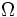$\Omega$, and is defined by: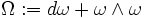$\Omega := d\omega + \omega \wedge \omega$

Here,$\omega$ is a matrix of connection forms.

### In the linear case

In the special case where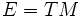$E = TM$ (the case of a linear connection) we get that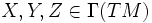$X,Y, Z \in \Gamma(TM)$. We can thus think of this map as a (1,3)-tensor because it takes as input three vector fields and outputs one vector field.

## Properties

### Tensoriality

Further information: Curvature is tensorial

The curvature is tensorial in all three arguments. This is best proved by proving$C^\infty$-linearity in all arguments, via a computation.

### Antisymmetry

Further information: Curvature is antisymmetric in first two variables

We have the following identity:$R(X,Y) = -R(Y,X)$